Bisector of an angle

Chapter 14 Class 6 Practical Geometry
Concept wise

Here, we will draw 75° using protractor.

To draw 75° using compass, please check Ex 11.1, 4 ( i ) of Class 9 NCERT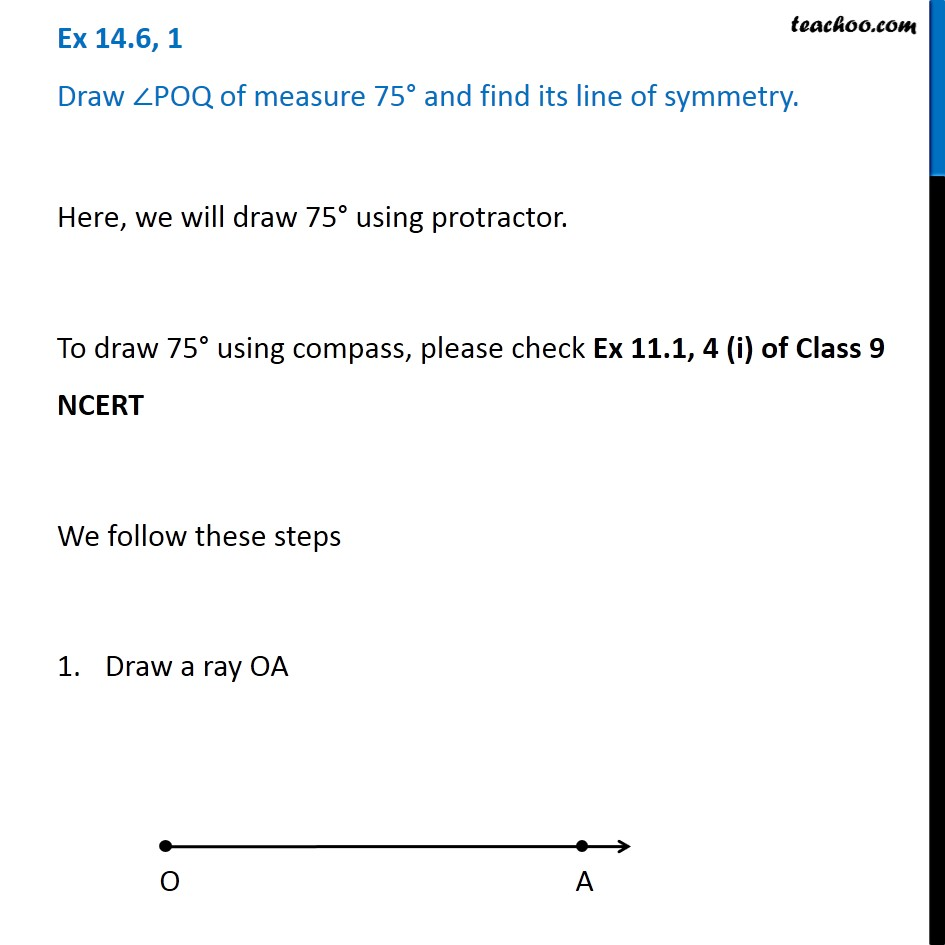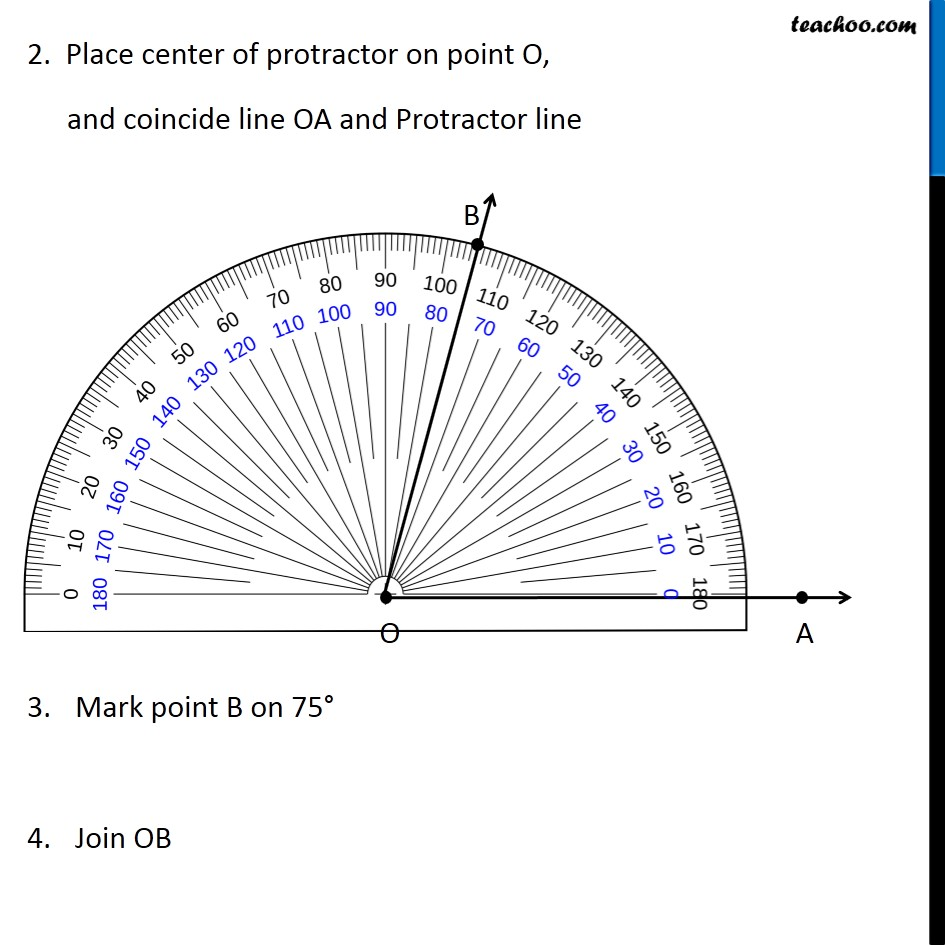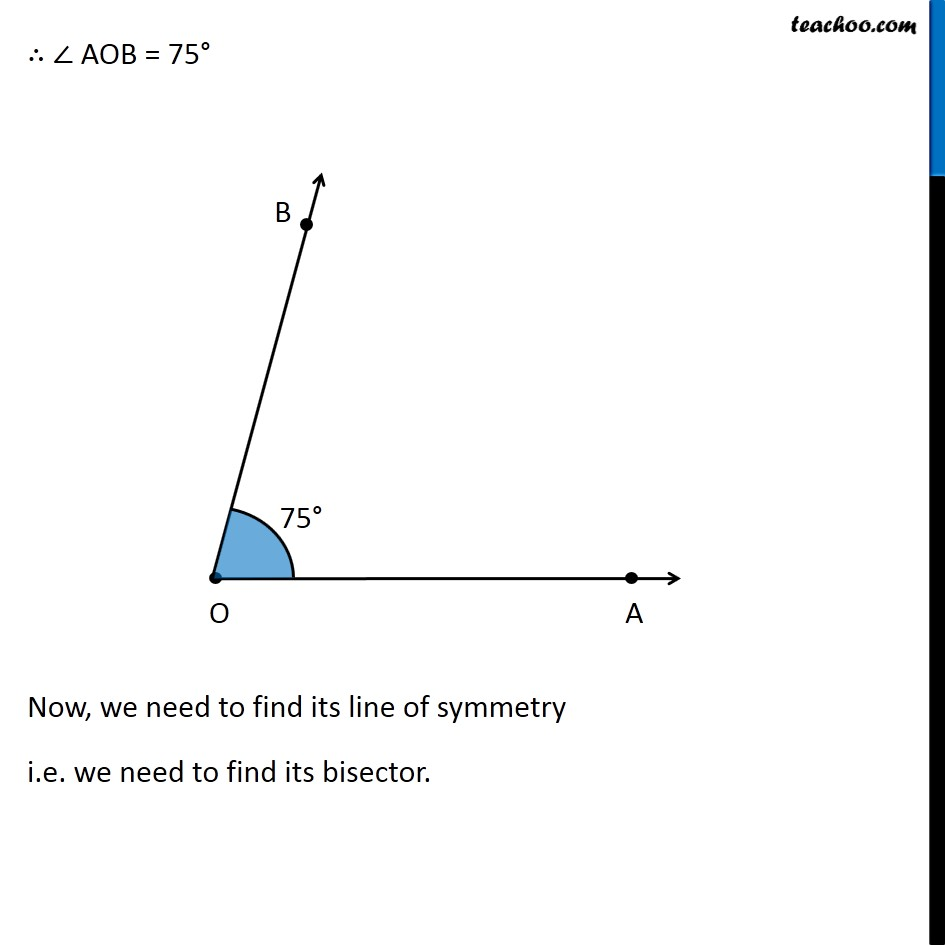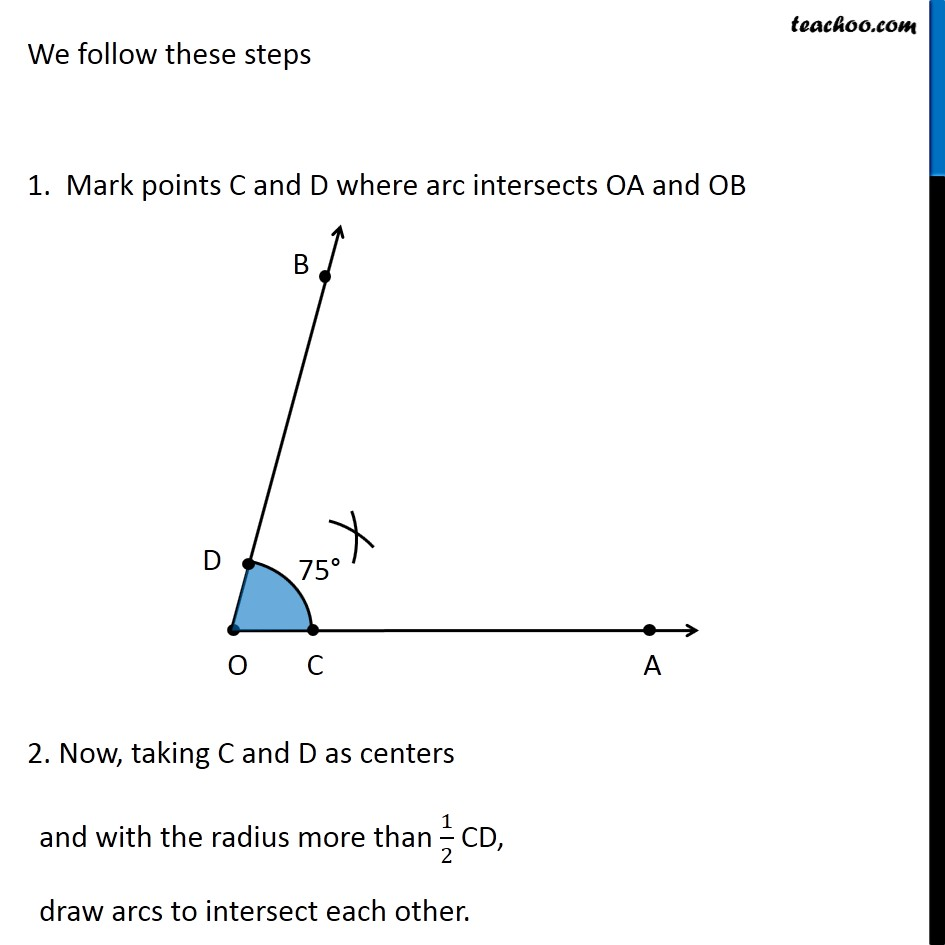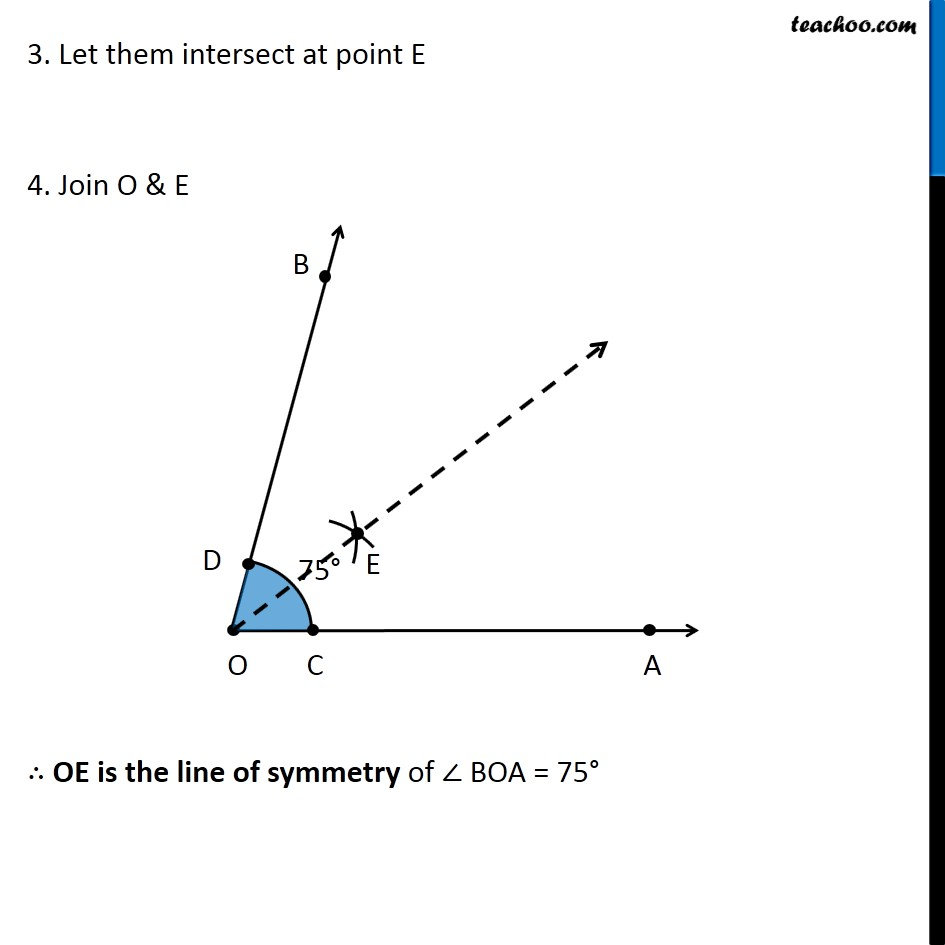Introducing your new favourite teacher - Teachoo Black, at only ₹83 per month

### Transcript

Ex 14.6, 1 Draw ∠POQ of measure 75° and find its line of symmetry. Here, we will draw 75° using protractor. To draw 75° using compass, please check Ex 11.1, 4 (i) of Class 9 NCERT We follow these steps Draw a ray OA 2. Place center of protractor on point O, and coincide line OA and Protractor line 3. Mark point B on 75° 4. Join OB ∴ ∠ AOB = 75° Now, we need to find its line of symmetry i.e. we need to find its bisector. We follow these steps 1. Mark points C and D where arc intersects OA and OB 2. Now, taking C and D as centers and with the radius more than 1/2 CD, draw arcs to intersect each other. 3. Let them intersect at point E 4. Join O & E ∴ OE is the line of symmetry of ∠ BOA = 75°